# TSA Confidence Limits VI

LabVIEW 2014 Advanced Signal Processing Toolkit Help

Edition Date: June 2014

Part Number: 372656C-01

»View Product InfoDownload Help (Windows Only)

Owning Palette: Statistical Analysis VIs

Computes confidence limits of the mean and standard deviation values of the univariate or multivariate (vector) time series. Wire data to the Xt input to determine the polymorphic instance to use or manually select the instance.

Use the pull-down menu to select an instance of this VI.

 Select an instance TSA Confidence Limits (Waveform)TSA Confidence Limits (Array)TSA Vector Confidence Limits (Waveform)TSA Vector Confidence Limits (Array)

## TSA Confidence Limits (Waveform)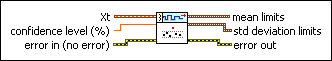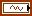Xt specifies the univariate time series.confidence level specifies the level of confidence, as a percentage, to use in computing the confidence limits of the mean and standard deviation values. The default is 95.error in describes error conditions that occur before this node runs. This input provides standard error in functionality.mean limits returns the confidence limits of the mean value at the specified confidence level.upper returns the upper confidence limit of the mean value at the specified confidence level.lower returns the lower confidence limit of the mean value at the specified confidence level.std deviation limits returns the confidence limits of the standard deviation value at the specified confidence level.upper returns the upper confidence limit of the standard deviation value at the specified confidence level.lower returns the lower confidence limit of the standard deviation value at the specified confidence level.error out contains error information. This output provides standard error out functionality.

## TSA Confidence Limits (Array)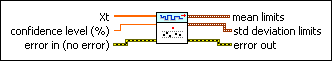Xt specifies the univariate time series.confidence level specifies the level of confidence, as a percentage, to use in computing the confidence limits of the mean and standard deviation values. The default is 95.error in describes error conditions that occur before this node runs. This input provides standard error in functionality.mean limits returns the confidence limits of the mean value at the specified confidence level.upper returns the upper confidence limit of the mean value at the specified confidence level.lower returns the lower confidence limit of the mean value at the specified confidence level.std deviation limits returns the confidence limits of the standard deviation value at the specified confidence level.upper returns the upper confidence limit of the standard deviation value at the specified confidence level.lower returns the lower confidence limit of the standard deviation value at the specified confidence level.error out contains error information. This output provides standard error out functionality.

## TSA Vector Confidence Limits (Waveform)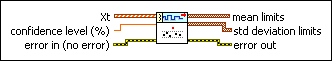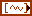Xt specifies the multivariate (vector) time series.confidence level specifies the level of confidence, as a percentage, to use in computing the confidence limits of the mean and standard deviation values. The default is 95.error in describes error conditions that occur before this node runs. This input provides standard error in functionality.mean limits returns the confidence limits of the mean value at the specified confidence level.upper returns the upper confidence limit of the mean value at the specified confidence level.lower returns the lower confidence limit of the mean value at the specified confidence level.std deviation limits returns the confidence limits of the standard deviation value at the specified confidence level.upper returns the upper confidence limit of the standard deviation value at the specified confidence level.lower returns the lower confidence limit of the standard deviation value at the specified confidence level.error out contains error information. This output provides standard error out functionality.

## TSA Vector Confidence Limits (Array)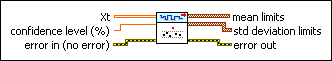Xt specifies the multivariate (vector) time series. Each column of the 2D array represents a vector at certain time.confidence level specifies the level of confidence, as a percentage, to use in computing the confidence limits of the mean and standard deviation values. The default is 95.error in describes error conditions that occur before this node runs. This input provides standard error in functionality.mean limits returns the confidence limits of the mean value at the specified confidence level.upper returns the upper confidence limit of the mean value at the specified confidence level.lower returns the lower confidence limit of the mean value at the specified confidence level.std deviation limits returns the confidence limits of the standard deviation value at the specified confidence level.upper returns the upper confidence limit of the standard deviation value at the specified confidence level.lower returns the lower confidence limit of the standard deviation value at the specified confidence level.error out contains error information. This output provides standard error out functionality.

## TSA Confidence Limits Details

### Confidence limits of the mean

This VI calculates confidence limits of the mean according to a test of the t distribution. If a time series satisfies the normal distribution with mean m and variance s2, the statistical value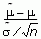satisfies t(n–1) distribution, where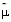is the estimation of m,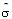is the estimation of s, and n is the number of univariate time series Xt.

Given the confidence level a,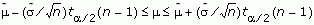### Confidence limits of the standard deviation

This VI calculates confidence limits of the standard deviation according to a test of c distribution. If a time series satisfies normal distribution with mean m and variance s2, then the statistical valuesatisfies c2(n–1) distribution, whereis the estimate of s, and n is the number of univariate time series Xt.

Given the confidence level a,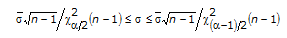### Reference:

NIST/SEMATECH e-Handbook of Statistical Methods, http://www.itl.nist.gov/div898/handbook, 2005.

## Example

Refer to the Series Statistical Analysis VI in the labview\examples\Time Series Analysis\TSAGettingStarted directory for an example of using the TSA Confidence Limits VI.